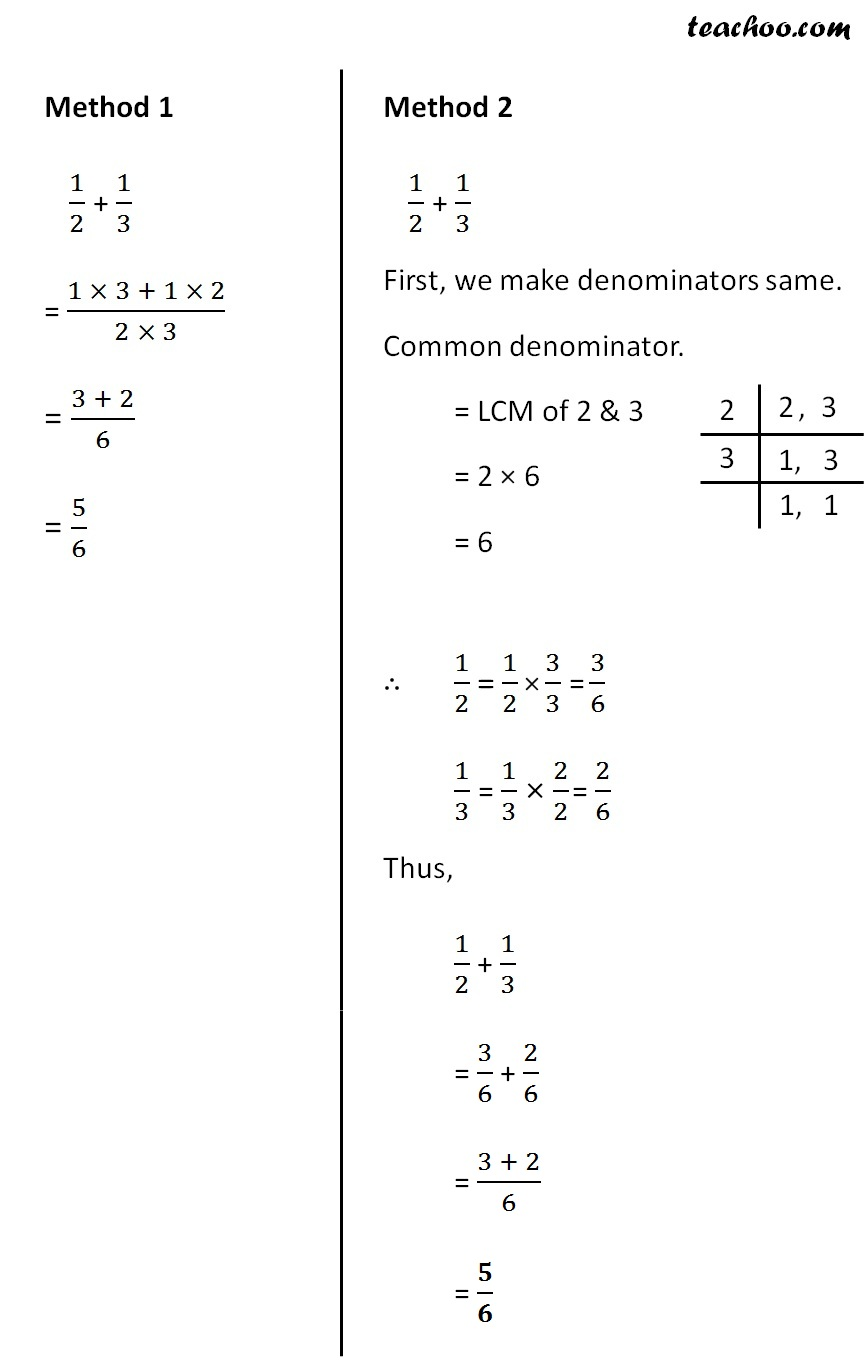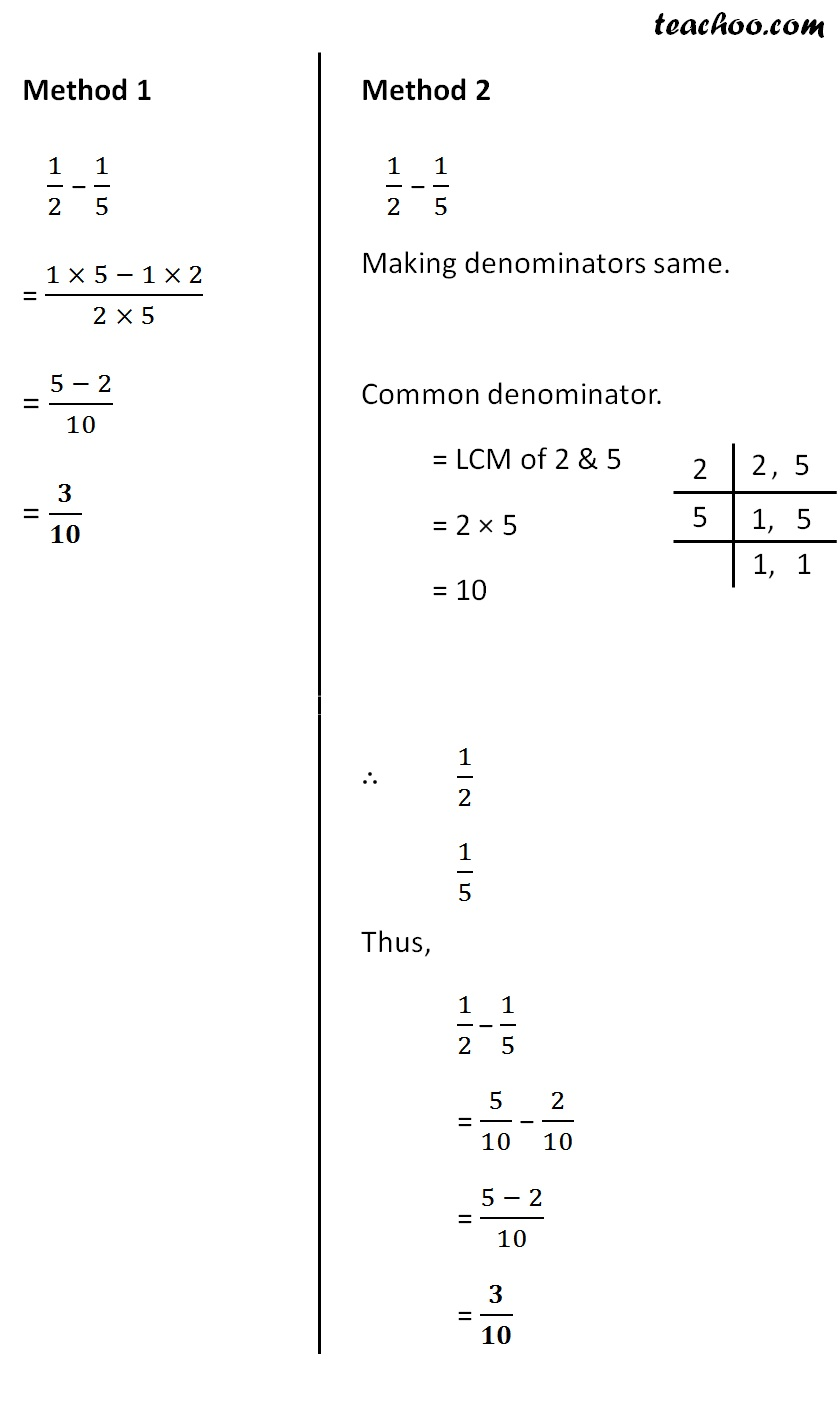Addition and Subtraction of Unlike Fractions

Chapter 7 Class 6 Fractions
Concept wise

Unlike fractions are those fractions where denominators are different

Eg: 1/2, 1/3, 1/5, 1/8

We use two methods to solve thisSubtract 1/2 & 1/5

1/2 − 1/5Get live Maths 1-on-1 Classs - Class 6 to 12

### Transcript

Adding & Subtracting Unlike FractionsUnlike fractions are those fractions where denominators are different Eg: 1/2, 1/3, 1/5, 1/8 Add 𝟏/𝟐 & 𝟏/𝟑 We use two methods to solve this Method 1 1/2 + 1/3 = (1 × 3 + 1 × 2)/(2 × 3) = (3 + 2)/6 = 5/6 Method 2 1/2 + 1/3 First, we make denominators same. Common denominator. = LCM of 2 & 3 = 2 × 6 = 6 ∴ 1/2 = 1/3 Thus, 1/2 + 1/3 = 3/6 + 2/6 = (3 + 2)/6 = 𝟓/𝟔 Subtract 𝟏/𝟐 & 𝟏/𝟓 1/2 − 1/5 Method 1 1/2 − 1/5 = (1 × 5 − 1 × 2)/(2 × 5) = (5 − 2)/6 = 𝟑/𝟏𝟎 Method 2 1/2 − 1/5 Making denominators same. Common denominator. = LCM of 2 & 5 = 2 × 5 = 10 ∴ 1/2 1/5 Thus, 1/2 − 1/5 = 5/10 − 2/10 = (5 − 2)/10 = 𝟑/𝟏𝟎